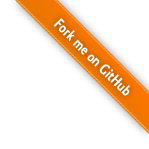# NLP之——Word2Vec详解

## Statistical Language Model

$S=w_1, w_2, ... , w_T$

$P(S)=P(w_1, w_2, ..., w_T)=\prod_{t=1}^Tp(w_t|w_1, w_2, ..., w_{t-1})$

$p(w_t|w_1,w_2,...,w_{t-1})$

$p(w_t|w_1, w_2, ..., w_{t-1}) \approx p(w_t|w_{t-n+1}, ..., w_{t-1})$

## Distributed Representation

VSM是基于一种Statistical Semantics Hypothesis：语言的统计特征隐藏着语义的信息（Statistical pattern of human word usage can be used to figure out what people mean）。例如，两篇具有相似词分布的文档可以被认为是有着相近的主题。这个Hypothesis有很多衍生版本。其中，比较广为人知的两个版本是Bag of Words HypothesisDistributional Hypothesis。前者是说，一篇文档的词频（而不是词序）代表了文档的主题；后者是说，上下文环境相似的两个词有着相近的语义。后面我们会看到，word2vec算法也是基于Distributional的假设。

## Neural Network Language Model

NNLM模型的基本思想可以概括如下：

1. 假定词表中的每一个word都对应着一个连续的特征向量；
2. 假定一个连续平滑的概率模型，输入一段词向量的序列，可以输出这段序列的联合概率；
3. 同时学习词向量的权重和概率模型里的参数。1. 首先是一个线性的Embedding层。它将输入的N−1个one-hot词向量，通过一个共享的D×V的矩阵C，映射为N−1个分布式的词向量（distributed vector）。其中，V是词典的大小，D是Embedding向量的维度（一个先验参数）。C矩阵里存储了要学习的word vector。

2. 其次是一个简单的前向反馈神经网络g。它由一个tanh隐层和一个softmax输出层组成。通过将Embedding层输出的N−1个词向量映射为一个长度为V的概率分布向量，从而对词典中的word在输入context下的条件概率做出预估：

$p(w_i|w_1,w_2,...,w_{t-1}) \approx f(w_i, w_{t-1}, ..., w_{t-n+1}) = g(w_i, C(w_{t-n+1}), ..., C(w_{t-1}))$

$L(\theta)=\frac{1}{T}\sum_t{\log{f(w_t, w_{t-1}, ..., w_{t-n+1})}}+R(\theta)$

## CBoW & Skip-gram Model

1. 用一个简单模型训练出连续的词向量；
2. 基于词向量的表达，训练一个连续的Ngram神经网络模型。
而NNLM模型的计算瓶颈主要是在第二步。

Mikolov是这么想的，也是这么做的。他在2013年一口气推出了两篇paper，并开源了一款计算词向量的工具——至此，word2vec横空出世，主角闪亮登场。

1. 移除前向反馈神经网络中非线性的hidden layer，直接将中间层的Embedding layer与输出层的softmax layer连接；
2. 忽略上下文环境的序列信息：输入的所有词向量均汇总到同一个Embedding layer；
3. 将Future words纳入上下文环境CBoW模型依然是从context对target word的预测中学习到词向量的表达。反过来，我们能否从target word对context的预测中学习到word vector呢？答案显然是可以的：$p(w_o|w_i)=\frac{e^{U_o \cdot V_i}}{\sum_j{e^{U_j \cdot V_i}}}$

### Hierarchical Softmax

$p(v|context)=\prod_{i=1}^m{p(b_i(v)|b_1(v), ..., b_{i-1}(v), context)}$

$p(w_t \in D_1|context)=\frac{1}{1+e^{-U_{D_{root}} \cdot V_{w_t}}}$

$p(w_t|context)=p(D_1=1|context)p(D_2=0|D_1=1)\dots p(w_t|D_k=1)$

### Negative Sampling

$J(\theta)=-\frac{1}{T}\sum_{t=1}^T{\sum_{-c \leq j \leq c, j \neq 0}{\log p(w_{t+j}|w_t)}}$

$p(D=1|w, context)=\frac{p(w|context)}{p(w|context)+kp_n(w)}=\sigma (\log p(w|context) - \log kp_n(w))$

$p(D=1|w_o, w_i)=\sigma (U_o \cdot V_i)$

$J(\theta) = \log \sigma(U_o \cdot V_i) + \sum_{j=1}^k{E_{w_j \sim p_n(w)}[\log \sigma(- U_j \cdot V_i)]}$

$p_{discard}(w) = 1 - \sqrt{\frac{t}{f(w)}}$

## Beyond the Word Vector

13年word2vec模型横空出世后，人们最津津乐道的是它学到的向量在语义和语法相似性上的应用——尤其是这种相似性居然对数学上的加减操作有意义！最经典的一个例子是，$v("King") - v("Man") + v("Woman") = v("Queen")$。然而，这种例子似乎并没有太多实际的用途。

### Machine Translation

$J(W)=\sum_{i=1}^n{||Wx_i - z_i||^2}$

## Word Embedding

Word embedding最早出现于Bengio在03年发表的开创性文章中。通过嵌入一个线性的投影矩阵（projection matrix），将原始的one-hot向量映射为一个稠密的连续向量，并通过一个语言模型的任务去学习这个向量的权重。这一思想后来被广泛应用于包括word2vec在内的各种NLP模型中。

Word Embedding的训练方法大致可以分为两类：一类是无监督或弱监督的预训练；一类是端对端（end to end）的有监督训练。

Word Embedding的另一个研究方向是在更高层次上对Sentence的Embedding向量进行建模。

: Mikolov, T., Chen, K., Corrado, G., & Dean, J. (2013, January 17). Efficient Estimation of Word Representations in Vector Space. arXiv.org.

: Mikolov, T., Sutskever, I., Chen, K., Corrado, G., & Dean, J. (2013, October 17). Distributed Representations of Words and Phrases and their Compositionality. arXiv.org.

: Bengio, Y., Ducharme, R., Vincent, P., & Janvin, C. (2003). A neural probabilistic language model. The Journal of Machine Learning Research, 3, 1137–1155.

: Turney, P. D., & Pantel, P. (2010). From frequency to meaning: vector space models of semantics. Journal of Artificial Intelligence Research, 37(1).

: Morin, F., & Bengio, Y. (2005). Hierarchical Probabilistic Neural Network Language Model. Aistats.

: Mnih, A., & Kavukcuoglu, K. (2013). Learning word embeddings efficiently with noise-contrastive estimation, 2265–2273.

: Mikolov, T., Karafiát, M., Burget, L., & Cernocký, J. (2010). Recurrent neural network based language model. Interspeech.

: Mikolov, T., Yih, W., & Zweig, G. (2013). Linguistic Regularities in Continuous Space Word Representations. Hlt-Naacl.

: Mikolov, T., Le, Q. V., & Sutskever, I. (2013, September 17). Exploiting Similarities among Languages for Machine Translation. arXiv.org.

: Collobert, R., Weston, J., Bottou, L., Karlen, M., Kavukcuoglu, K., & Kuksa, P. (2011). Natural Language Processing (Almost) from Scratch. Journal of Machine Learning Research, 12(Aug), 2493–2537.

: Barkan, O., & Koenigstein, N. (2016, March 14). Item2Vec: Neural Item Embedding for Collaborative Filtering. arXiv.org.

: Le, Q. V., & Mikolov, T. (2014, May 16). Distributed Representations of Sentences and Documents. arXiv.org.

posted @ 2018-06-28 19:29  郭耀华  阅读(95542)  评论(8编辑  收藏  举报